# Determining Whether A Worked Shift Was Morning, Afternoon Or Night In Microsoft Excel 2010

In this article, we will learn how to determine whether a worked shift was morning, afternoon or night in Microsoft Excel 2010.

To find a formula to determine whether an employee worked in morning, afternoon or night shift, we will use IF & LOOKUP functions to get the output.

The IF function checks if a condition you specify is TRUE or FALSE. If the condition returns TRUE then it returns preset value, and if ion returns FALSE then it returns another preset value.

Syntax = IF(logical_test,value_if_true,value_if_false)

logical_test: Logical test will test the condition or criteria, if condition meets then it returns the preset value, and if the condition does not meet then it returns another preset value.

value_if_true: The value that you want to be returned if this argument returns TRUE.

value_if_false: The value that you want to be returned if this argument returns FALSE

LOOKUP: Looks up a value either from a one-row or one-column range or from an array. Provided for backward compatibility.

Syntax =LOOKUP(lookup_value,lookup_vector,result_vector)

lookup_value: A value that LOOKUP function searches for in the lookup vector.

lookup_vector: A range that contains only one row or one column.

Note: Values in lookup_vector must be placed in ascending order (1,2,3 or a,b,c). Otherwise lookup function will return the error value.

result_vector: This is optional. A range that contains only one row or column. The result_vector argument must be of the same size as lookup_vector.

Let us take an example:

• Column A contains Login time.
• We need a formula to use the login times from column A to determine whether a person was starting a morning, an afternoon, or a night shift.
• For times before 12:00, the formula should return “Morning Shift”.
• For times between 12:00 and 16:00, the formula should return “Afternoon Shift”.
• For times after 16:00, the formula should return “Night Shift”.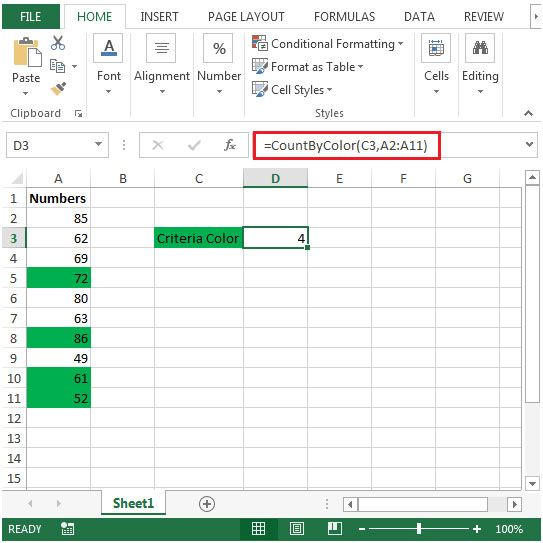• In cell B2, the formula would be
=IF(A2>=2/3,"Night",IF(A2>=0.5,"Afternoon","Morning"))&" Shift"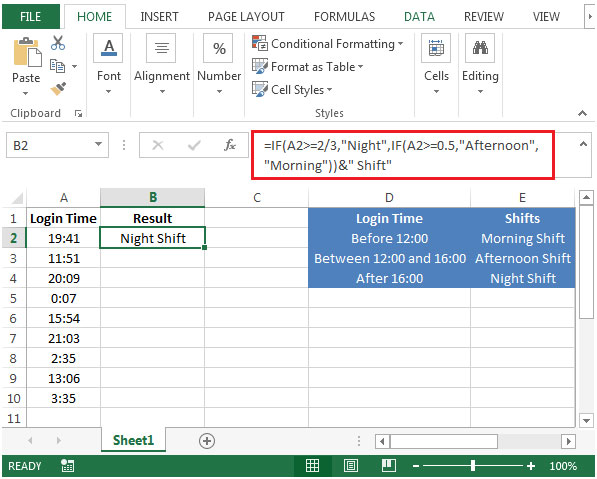• Copying down the formula from cell B2 to range B3:B10, we will get the desired result.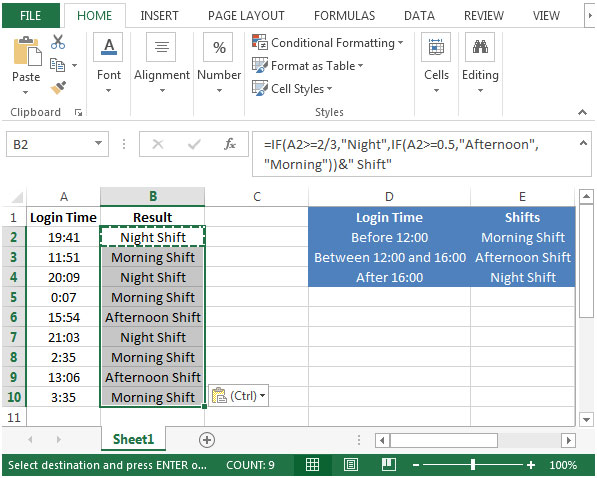The second method is to use LOOKUP function.

• In cell C2 the formula would be
=LOOKUP(HOUR(A2),{0,12,16},{"Morning","Afternoon","Night"})&" Shift"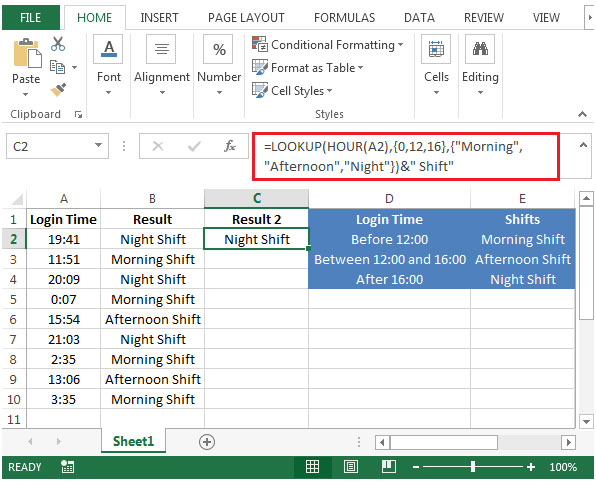• Copying down the formula from cell C2 to range C3:C10, we will get the desired result.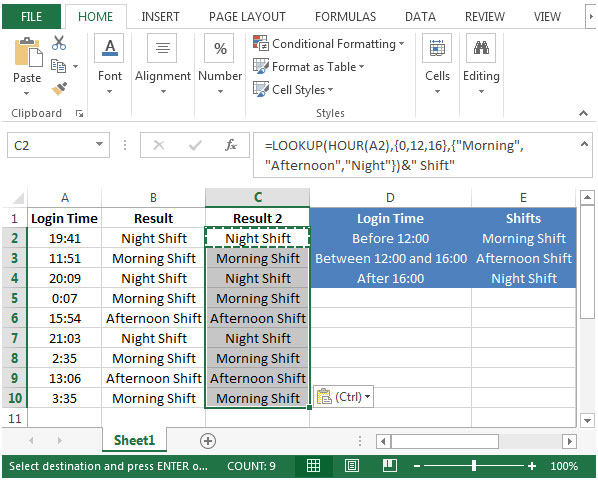Terms and Conditions of use

The applications/code on this site are distributed as is and without warranties or liability. In no event shall the owner of the copyrights, or the authors of the applications/code be liable for any loss of profit, any problems or any damage resulting from the use or evaluation of the applications/code.